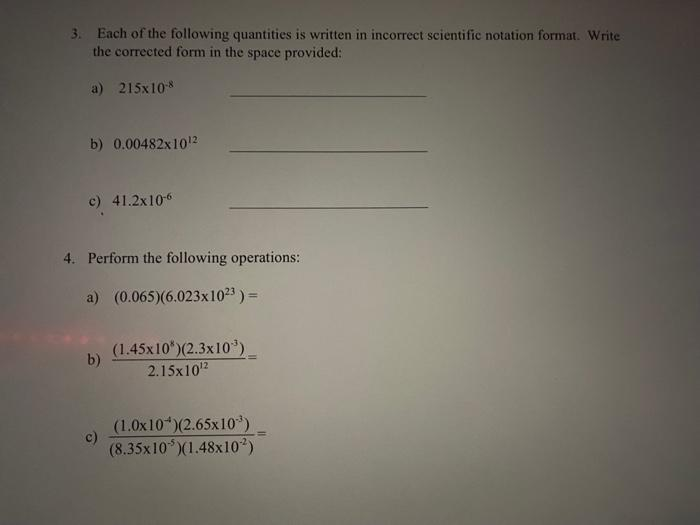Home / Expert Answers / Chemistry / 1-write-each-of-the-following-in-scientific-notation-a-180000000-b-0-000143-2-which-numb-pa656

# (Solved): 1. Write each of the following in scientific notation: a) 180000000 b) $$0.000143$$ 2. Which numb ...1. Write each of the following in scientific notation: a) 180000000 b) $$0.000143$$ 2. Which number in each of the following pairs is larger? (Circle your choice) a) $$4.9 \times 10^{-3}$$ or $$5.5 \times 10^{-6}$$ b) $$2.5 \times 10^{4} \quad$$ or $$1.2 \times 10^{6}$$ c) $$1250 \quad$$ or $$3.4 \times 10^{2}$$ 3. Each of the following quantities is written in incorrect scientific notation format. Write the corrected form in the space provided: a) $$215 \times 10^{-8}$$ b) $$0.00482 \times 10^{12}$$ c) $$41.2 \times 10^{-6}$$ 4. Perform the following operations: a) $$(0.065)\left(6.023 \times 10^{23}\right)=$$ b) $$\frac{\left(1.45 \times 10^{8}\right)\left(2.3 \times 10^{-3}\right)}{2.15 \times 10^{12}}=$$ c) $$\frac{\left(1.0 \times 10^{-4}\right)\left(2.65 \times 10^{-3}\right)}{\left(8.35 \times 10^{-5}\right)\left(1.48 \times 10^{-2}\right)}=$$

We have an Answer from Expert